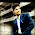## Saturday, 1 August 2015

### Quick Select -Finding K'th Largest

Quick select is one of very popular algorithm used to find k'th largest element in a list. It can be use to find median or finding k'th smallest element etc.

Finding K'th largest element looks easy , we just need to sort & get K'th largest . But here we are dealing with time so we need fastest way to do this
Time complexity of Quick Select (average case) =O(n)
Quick Select is basically modified version of Quick sort. In Quick Sort we need to sort all element but Quick select just want to know pivot value that divide array in given ratio.

let a example we have 10 different number in array  & we want to find 3rd largest element.(we  assume 0..9 index array) then in sorted array its position should be (10-3)th Index.

So basically we have to do is find a pivot that divide array in 2 group ,left group contain all element less then pivot and right group contain all element greater than pivot .
So if we want 3rd largest element then pivot index will be (n-3) and data at that index is third largest data .

If you did not Understand than probability you don't know Quick sort . I strongly Recommend first to understand Quick sort. Even though you did't get it then follow our code .

## Quick Select -Find K'th largest element

1. //Quick Selelct
2. //very useful for
3. //Find kth largest or smallest element
4. //find median
5. //O(n)
6. //this program demonstrate to find K'th largest from given list
7. //assuming all are different numbers
8. #include<iostream>
9. #include<vector>
10. using namespace std;
11. int Quick_Select(vector<int> &arr,int p,int r,int index_of_kth_largest);
12. int partition(vector<int> &arr,int p,int r,int pivot);
13. int main(){
14.         vector<int> arr;
15.         int n,k;
16.         cout<<"Enter No of element (n) :";
17.         cin>>n;
18.         cout<<"Enter Value of k :";
19.         cin>>k;
20.         cout<<"Enter array element :"<<endl;
21.         int key;
22.         for(int i=0;i<n;i++){
23.                 cin>>key;
24.                 arr.push_back(key);
25.         }
26.         int kth=Quick_Select(arr,0,n-1,n-k);
27.         cout<<"K'th largest number is "<<kth;
28.         return 0;
29. }
30. int Quick_Select(vector<int> &arr,int p,int r,int index_of_kth_largest){
31.         //We are basically finding situation when pivote == index_of_kth_largest
32.         //then all elemnt less than that is on left side and all element greter than that is in right side
33.         if(index_of_kth_largest==r)
34.                 return arr[r];
35.         int pivotI;
36.         while(1){
37.                 pivotI=(p+r)/2;
38.                 pivotI=partition(arr,p,r,pivotI);
39.                 if(pivotI== index_of_kth_largest)
40.                         return arr[pivotI];
41.                 //make sure to chhose proper sub arr where index=index_of_kth_largest reside
42.                 if(pivotI <index_of_kth_largest)
43.                         p=pivotI+1;
44.                 if(pivotI>index_of_kth_largest)
45.                         r=pivotI-1;
46.         }
47. }
48. int partition(vector<int> &arr,int p,int r,int pivotIndex){
49.         int i=p-1;
50.         //swap and move pivote  data to end
51.         int temp;
52.         int pivot=arr[pivotIndex];
53.         temp=arr[pivotIndex];
54.         arr[pivotIndex]=arr[r];
55.         arr[r]=temp;
56.
57.         //arrange arr such that all elemnt below pivote come to left side of pivotIndex
58.         //and all elemnt greter than pivot come right side of pivotIndex
59.         for(int j=p;j<r;j++){
60.                 if(pivot<=arr[j]){
61.                         i++;
62.                         temp=arr[i];
63.                         arr[i]=arr[j];
64.                         arr[j]=temp;
65.                 }
66.         }
67.         i++;
68.         temp=arr[i];
69.         arr[i]=arr[r];
70.         arr[r]=temp;
71.         return i;
72. }

#### 1 comment:

1.I really appreciate information shared above. It’s of great help. If someone want to learn Online (Virtual) instructor lead live training in Data Science with Python , kindly contact us http://www.maxmunus.com/contact
MaxMunus Offer World Class Virtual Instructor led training on TECHNOLOGY. We have industry expert trainer. We provide Training Material and Software Support. MaxMunus has successfully conducted 100000+ trainings in India, USA, UK, Australlia, Switzerland, Qatar, Saudi Arabia, Bangladesh, Bahrain and UAE etc.